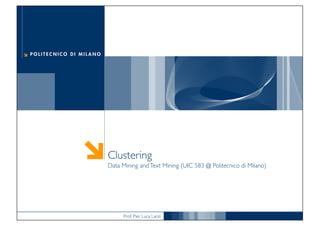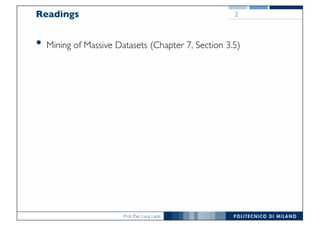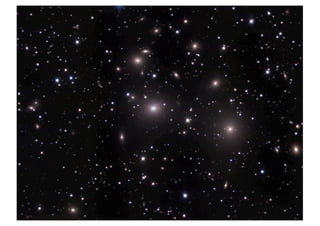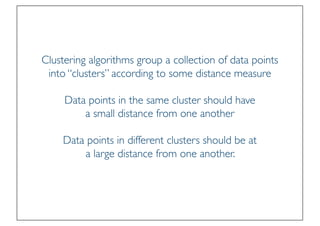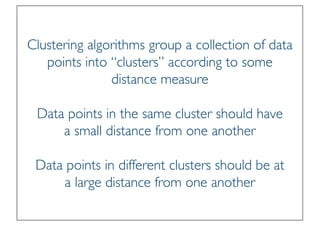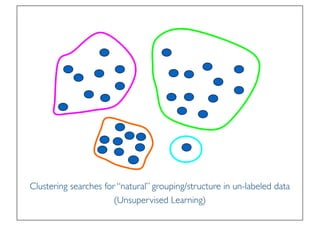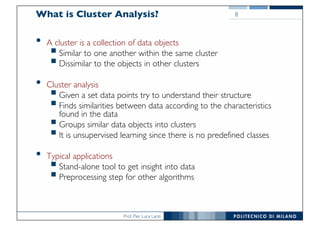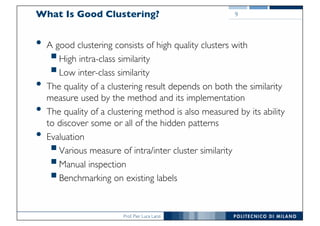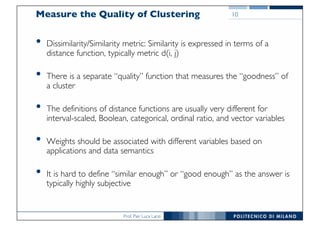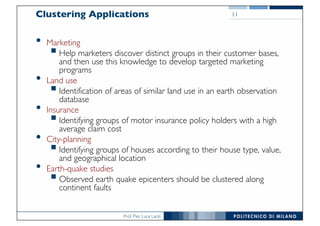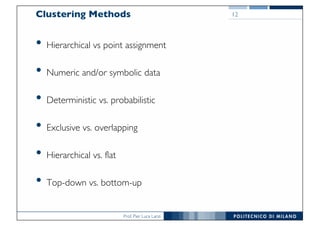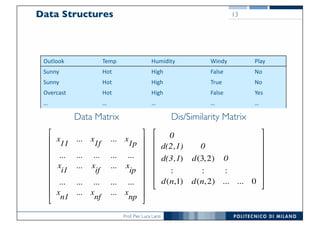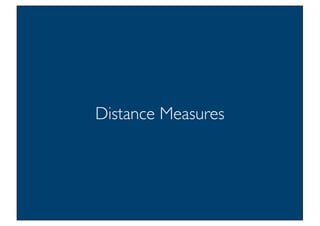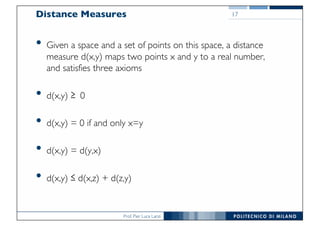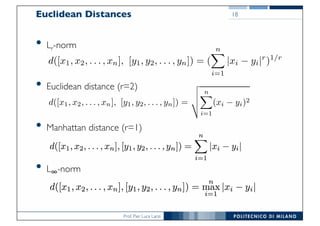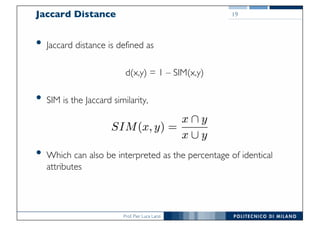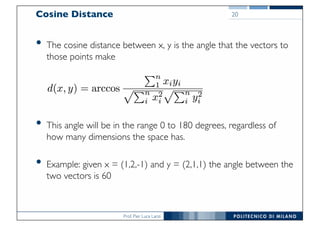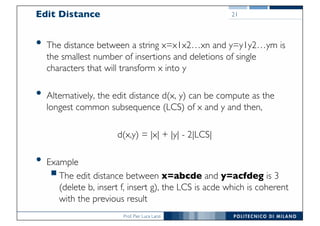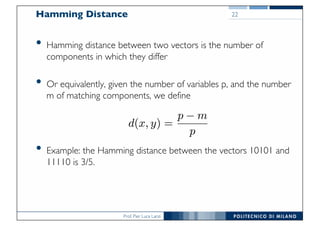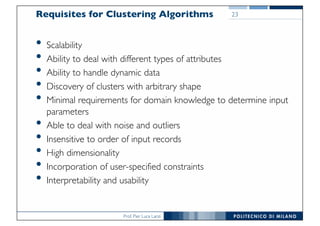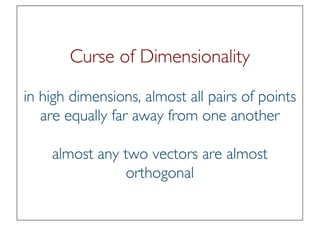1 de 22

### DMTM Lecture 11 Clustering

1. Prof. Pier Luca Lanzi Clustering Data Mining andText Mining (UIC 583 @ Politecnico di Milano)
2. Prof. Pier Luca Lanzi Readings • Mining of Massive Datasets (Chapter 7, Section 3.5) 2
3. Prof. Pier Luca Lanzi
4. Prof. Pier Luca Lanzi 4
5. Prof. Pier Luca Lanzi Clustering algorithms group a collection of data points into “clusters” according to some distance measure Data points in the same cluster should have a small distance from one another Data points in different clusters should be at a large distance from one another.
6. Prof. Pier Luca Lanzi Clustering algorithms group a collection of data points into “clusters” according to some distance measure Data points in the same cluster should have a small distance from one another Data points in different clusters should be at a large distance from one another
7. Prof. Pier Luca Lanzi Clustering searches for “natural” grouping/structure in un-labeled data (Unsupervised Learning)
8. Prof. Pier Luca Lanzi What is Cluster Analysis? • A cluster is a collection of data objects §Similar to one another within the same cluster §Dissimilar to the objects in other clusters • Cluster analysis §Given a set data points try to understand their structure §Finds similarities between data according to the characteristics found in the data §Groups similar data objects into clusters §It is unsupervised learning since there is no predefined classes • Typical applications §Stand-alone tool to get insight into data §Preprocessing step for other algorithms 8
9. Prof. Pier Luca Lanzi What Is Good Clustering? • A good clustering consists of high quality clusters with §High intra-class similarity §Low inter-class similarity • The quality of a clustering result depends on both the similarity measure used by the method and its implementation • The quality of a clustering method is also measured by its ability to discover some or all of the hidden patterns • Evaluation §Various measure of intra/inter cluster similarity §Manual inspection §Benchmarking on existing labels 9
10. Prof. Pier Luca Lanzi Measure the Quality of Clustering • Dissimilarity/Similarity metric: Similarity is expressed in terms of a distance function, typically metric d(i, j) • There is a separate “quality” function that measures the “goodness” of a cluster • The definitions of distance functions are usually very different for interval-scaled, Boolean, categorical, ordinal ratio, and vector variables • Weights should be associated with different variables based on applications and data semantics • It is hard to define “similar enough” or “good enough” as the answer is typically highly subjective 10
11. Prof. Pier Luca Lanzi Clustering Applications • Marketing §Help marketers discover distinct groups in their customer bases, and then use this knowledge to develop targeted marketing programs • Land use §Identification of areas of similar land use in an earth observation database • Insurance §Identifying groups of motor insurance policy holders with a high average claim cost • City-planning §Identifying groups of houses according to their house type, value, and geographical location • Earth-quake studies §Observed earth quake epicenters should be clustered along continent faults 11
12. Prof. Pier Luca Lanzi Clustering Methods • Hierarchical vs point assignment • Numeric and/or symbolic data • Deterministic vs. probabilistic • Exclusive vs. overlapping • Hierarchical vs. flat • Top-down vs. bottom-up 12
13. Prof. Pier Luca Lanzi Data Structures 0 d(2,1) 0 d(3,1) d(3,2) 0 : : : d(n,1) d(n,2) ... ... 0 ! " # # # # # # \$ % & & & & & & Outlook Temp Humidity Windy Play Sunny Hot High False No Sunny Hot High True No Overcast Hot High False Yes … … … … … x 11 ... x 1f ... x 1p ... ... ... ... ... x i1 ... x if ... x ip ... ... ... ... ... x n1 ... x nf ... x np ! " # # # # # # # # \$ % & & & & & & & & Data Matrix 13 Dis/Similarity Matrix
14. Prof. Pier Luca Lanzi Distance Measures
15. Prof. Pier Luca Lanzi Distance Measures • Given a space and a set of points on this space, a distance measure d(x,y) maps two points x and y to a real number, and satisfies three axioms • d(x,y) ≥ 0 • d(x,y) = 0 if and only x=y • d(x,y) = d(y,x) • d(x,y) ≤ d(x,z) + d(z,y) 17
16. Prof. Pier Luca Lanzi Euclidean Distances 18 There are other distance measures that have been used for Euclidean spa For any constant r, we can deﬁne the Lr-norm to be the distance measur deﬁned by: d([x1, x2, . . . , xn], [y1, y2, . . . , yn]) = ( n i=1 |xi − yi|r )1/r The case r = 2 is the usual L2-norm just mentioned. Another common dista measure is the L1-norm, or Manhattan distance. There, the distance betw wo points is the sum of the magnitudes of the diﬀerences in each dimens t is called “Manhattan distance” because it is the distance one would have • Lr-norm • Euclidean distance (r=2) • Manhattan distance (r=1) • L∞-norm 3.5.2 Euclidean Distances The most familiar distance measure is the one we normally think of as “dis- ance.” An n-dimensional Euclidean space is one where points are vectors of n eal numbers. The conventional distance measure in this space, which we shall efer to as the L2-norm, is deﬁned: d([x1, x2, . . . , xn], [y1, y2, . . . , yn]) = n i=1 (xi − yi)2 That is, we square the distance in each dimension, sum the squares, and take he positive square root. It is easy to verify the ﬁrst three requirements for a distance measure are atisﬁed. The Euclidean distance between two points cannot be negative, be- cause the positive square root is intended. Since all squares of real numbers are nonnegative, any i such that xi ̸= yi forces the distance to be strictly positive. On the other hand, if xi = yi for all i, then the distance is clearly 0. Symmetry ollows because (xi − yi)2 = (yi − xi)2 . The triangle inequality requires a good deal of algebra to verify. However, it is well understood to be a property of
17. Prof. Pier Luca Lanzi Jaccard Distance • Jaccard distance is defined as d(x,y) = 1 – SIM(x,y) • SIM is the Jaccard similarity, • Which can also be interpreted as the percentage of identical attributes 19
18. Prof. Pier Luca Lanzi Cosine Distance • The cosine distance between x, y is the angle that the vectors to those points make • This angle will be in the range 0 to 180 degrees, regardless of how many dimensions the space has. • Example: given x = (1,2,-1) and y = (2,1,1) the angle between the two vectors is 60 20
19. Prof. Pier Luca Lanzi Edit Distance • The distance between a string x=x1x2…xn and y=y1y2…ym is the smallest number of insertions and deletions of single characters that will transform x into y • Alternatively, the edit distance d(x, y) can be compute as the longest common subsequence (LCS) of x and y and then, d(x,y) = |x| + |y| - 2|LCS| • Example §The edit distance between x=abcde and y=acfdeg is 3 (delete b, insert f, insert g), the LCS is acde which is coherent with the previous result 21
20. Prof. Pier Luca Lanzi Hamming Distance • Hamming distance between two vectors is the number of components in which they differ • Or equivalently, given the number of variables p, and the number m of matching components, we define • Example: the Hamming distance between the vectors 10101 and 11110 is 3/5. 22
21. Prof. Pier Luca Lanzi Requisites for Clustering Algorithms • Scalability • Ability to deal with different types of attributes • Ability to handle dynamic data • Discovery of clusters with arbitrary shape • Minimal requirements for domain knowledge to determine input parameters • Able to deal with noise and outliers • Insensitive to order of input records • High dimensionality • Incorporation of user-specified constraints • Interpretability and usability 23
22. Prof. Pier Luca Lanzi Curse of Dimensionality in high dimensions, almost all pairs of points are equally far away from one another almost any two vectors are almost orthogonal